## 沙丘背风侧不同铁路结构形式对风沙环境的适应性分析 1)

*西部灾害与环境力学教育部重点实验室,兰州 730000

## ANALYSIS OF WIND-SAND MOVEMENT OVER SAND DUNE WITH DIFFERENT RAILWAY FORMS DOWNSTREAM 1)

Wang Wenbo*,, Huang Ning*, Dun Hongchao,*,2)

*Key Laboratory of Mechanics on Disaster and Environment in Western China,the Ministry of Education of China, Lanzhou 730000, China

China Railway First Survey and Design Institute Group Ltd, Lanzhou 730000, China

 基金资助: 1)国家重点研究开发项目.  2016YFC0500901国家自然科学基金项目.  11772143国家自然科学基金项目.  41730644中国科学院寒区旱区环境与工程研究所科技服务网络规划项目.  HHS-TSS-STS-1504中央高校基金.  lzujbky-2020-cd06

Received: 2020-02-15   Accepted: 2020-04-28   Online: 2020-05-18Abstract

When the railway goes through the windy desert area, the sand hazard endangers the railway engineering and normal operation of trains, and a suitable railway form design will reduce the hazard of accumulation of sand particles on railway. In this paper, taking Shashangou area of Dunhuang to Golmud railway as the research object, the multiphase flow method is used to simulate the wind sand movement over a sand dune with a railway in the reverse region, and the influence of wind sand movement on the railway subgrade engineering and bridge engineering located on the leeward slope of sand dunes is studied respectively. The main results of this work show that: The subgrade project significantly reduces the wind speed and divides the reverse area behind the sand dune into two parts, and the diversion effect of the bridge project compresses the reverse area of the leeward slope of the dune; the ballast between the tracks increases the aerodynamic roughness of the railway surface, and there is a small amount of sand deposition between tracks and a huge sand accumulations in both side of the subgrade; the complex interaction between wind field and sand particles makes the accumulated mass profiles of sand particles present non-linear relationship, the wind sweep ability for sand particles on the surface of railway grows faster than the sand accumulation ability with the increasing friction velocity in the bridge case, and this phenomenon is opposite in the subgrade case. According to the conclusion, it can be seen that the bridge engineering is better than the subgrade engineering in preventing the railway from being damaged by wind sand movement. This work offers a theoretical support for the influence of wind sand movement on railway engineering, and provides a new research thought and tool for the railway engineering design in the future.

Keywords： Dunhuang Golmud railway ; wind-sand movement ; numerical simulation ; subgrade project ; bridge project

Wang Wenbo, Huang Ning, Dun Hongchao. ANALYSIS OF WIND-SAND MOVEMENT OVER SAND DUNE WITH DIFFERENT RAILWAY FORMS DOWNSTREAM 1). Chinese Journal of Theoretical and Applied Mechanics[J], 2020, 52(3): 680-688 DOI:10.6052/0459-1879-20-043

## 引言

### 图1Fig.1   Landform and railway bridge map of Shashangou railway

## 1 数值模拟方法

### 1.1 连续性方程

$\nabla \cdot \left( {\phi _{a} \rho _{a} U_{a} } \right) = 0$

$\nabla \cdot \left( {\phi _{s} \rho _{s} U_{s} } \right) = 0$

### 1.2 动量方程

$\dfrac{\partial }{\partial t}\left( {\phi _{a} \rho _{a} U_{a} } \right) + \nabla \cdot \left( {\phi _{a} \rho _{a} U_{a} U_{a} } \right) = \\ \qquad - \phi _{a} \nabla p + \nabla \tau _{a} + \phi _{a} \rho _{a} g - f_{sa}$

$\dfrac{\partial }{\partial t}\left( {\phi _{s} \rho _{s} U_{s} } \right) + \nabla \cdot \left( {\phi _{s} \rho _{s} U_{s} U_{s} } \right) = \\ \qquad - \phi _{s} \nabla p - \nabla p_{s} + \nabla \tau _{s} + \phi _{s} \rho _{s} g + f_{sa}$

${\pmb\tau}_{a} = \phi _{a} u_{a} \left( {\nabla U_{a} + \nabla U_{a}^{T} } \right) + \phi _{a} \left( {u'_{a} - \dfrac{2}{3}u_{a} } \right)\nabla U_{\rm a} {\pmb I}$
${\pmb\tau}_{s} = \phi _{s} u_{s} \left( {\nabla U_{s} + \nabla U_{s}^{T} } \right) + \phi _{s} \left( {u'_{s} - \dfrac{2}{3}u_{s} } \right)\nabla U_{s} {\pmb I}$

$C_{D} = \left\{ \!\! \begin{array}{ll} 24\left( {1 + 0.15Re^{0.687}} \right) / Re &(Re \leqslant 1000) \\ 0.44 & (Re > 1000) \end{array} \!\!\right.$

$Re$是相对运动雷诺数

$Re = \dfrac{u_{a} \left| {U_{r} } \right|d}{\rho _{a} }$

$p_{s} = \phi _{s} \rho _{s} \theta _{s} + 2\rho _{s} \left( {1 + e_{s} } \right)\phi _{s}^2 d_0 \theta _{s}$

$d_0 = \left[ {1 - \left( {\dfrac{\phi _{s} }{\phi _{s\max } }} \right)^{\tfrac{1}{3}}} \right]^{ - 1}$

$\theta _{s}$表示的沙粒的颗粒温度描述了沙粒随机运动的动能,其方程如下

$\begin{array}\babl \dfrac{3}{2}\left[ {\dfrac{\partial }{\partial t}\left( {\phi _{s} \rho _{s} \theta _{s} } \right) + \nabla \cdot \phi _{s} \rho _{s} \theta _{s} U_{s} } \right] = \\ \qquad \left( {1 - p_{s} I + \tau _{s} } \right)\nabla U_{s} + \nabla \cdot \left( {k_{\theta _{s} } \nabla \theta _{s} } \right) - \gamma _{\theta _{s} } + a_{\theta _{s} } \end{array}$

$\gamma _{\theta _{s} } = \dfrac{6.12\phi _{s} \rho _{s}^2 \theta _{s}^{\tfrac{3}{2}} d_0}{d\sqrt{\pi}}$

$u_{s} = u_{scol} + u_{skin}$
$u'_{s} = \dfrac{4}{3}\phi _{s} \rho _{s} dd_0 \left( {1 + e_{s} }\right)\left( {\dfrac{\theta _{s} }{\pi }} \right)^{\tfrac{1}{2}}$

$u_{scol} = \dfrac{4}{5}\phi _{s} \rho _{s} dd_0 \left( {1 + e_{s} } \right)\left( {\dfrac{\theta _{s} }{\pi }} \right)$
$u_{skin} = \dfrac{\phi _{s} \rho _{s} d\sqrt {\pi \theta _{s} } }{6\left( {3 - {e}_{s} } \right)}\left[ {1 + \dfrac{2}{5}\left( {1 + e_{s} } \right)\left( {3 - e_{s} } \right)\phi _{s} d_0 } \right]$

### 1.3 $k$-$\varepsilon$湍流模型

$\dfrac{\partial \left( {\phi _\alpha \rho _\alpha k_\alpha } \right)}{\partial t} + \dfrac{\partial \left( {\phi _\alpha \rho _\alpha k_\alpha U_{\alpha i} } \right)}{\partial x_i } = \\ \qquad \dfrac{\partial }{\partial x_j } \left[ {\left( {\mu + \dfrac{\mu _t }{\sigma_k }} \right)\dfrac{\partial k_\alpha } {\partial x_j }} \right] + G_{k _\alpha} - G_{ba} - \phi _\alpha \rho _\alpha \varepsilon _\alpha$
$\dfrac{\partial \left( {\phi _\alpha \rho _\alpha \varepsilon _\alpha } \right)}{\partial t} + \dfrac{\partial \left( {\phi _\alpha \rho _\alpha \varepsilon _\alpha U_{\alpha i} } \right)}{\partial x_i } = \\ \qquad \dfrac{\partial }{\partial x_j }\left[ {\left( {\mu + \dfrac{\mu _t }{\sigma _\varepsilon }} \right)\dfrac{\partial \varepsilon _\alpha }{\partial x_j }} \right] + C_{1\varepsilon } \dfrac{\varepsilon _\alpha }{k_\alpha }(G_{k _{\alpha }} + C_{3\varepsilon } G_{b _{\alpha }} ) -\\ \qquad C_{2\varepsilon } \phi _\alpha \rho _\alpha \dfrac{\varepsilon _\alpha ^2}{k_\alpha }$

### 图2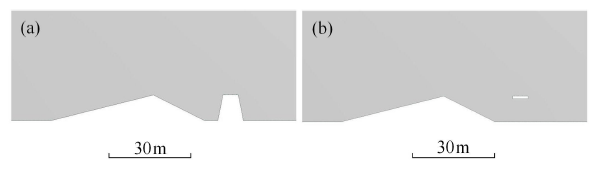Fig.2   Two kinds of typical design for railway in a leeside region of sand dune

### 图3Fig.3   The simulation domain and grid system used in subgrade designs computational

### 图4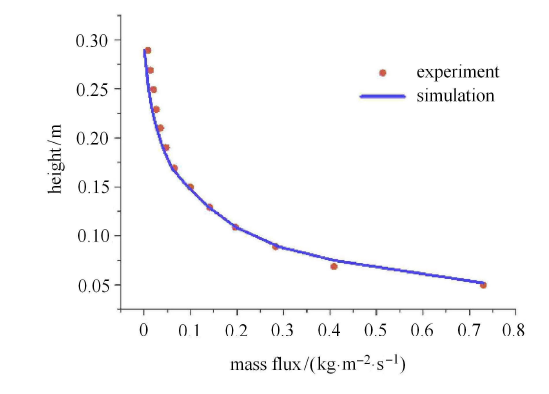Fig.4   The comparison of mass flux profile between simulation results and experiment results at the position of 8m downstream of the inlet

## 2 模拟结果及讨论

### 图5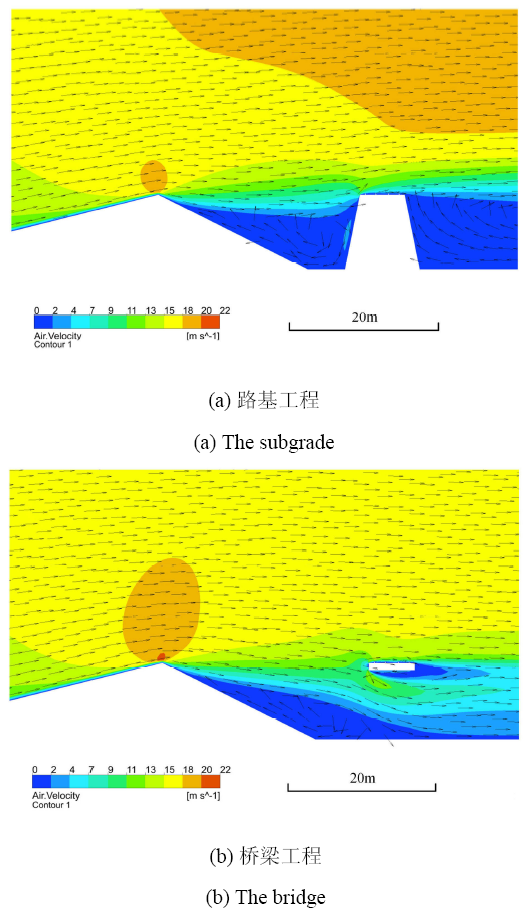Fig.5   The velocity nephogram and vector diagram of wind field when the friction velocity is 0.5m/s

### 图6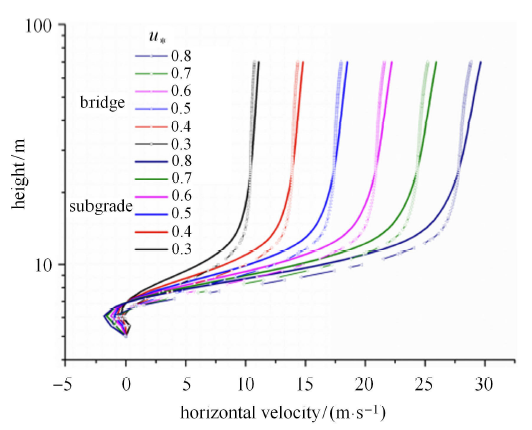Fig.6   The horizontal velocity profiles of wind field with height in the middle of sand dune leeside at the position $x =150$m. Straight lines are the profiles in the subgrade design, and the shape lines are in the bridge design

### 图7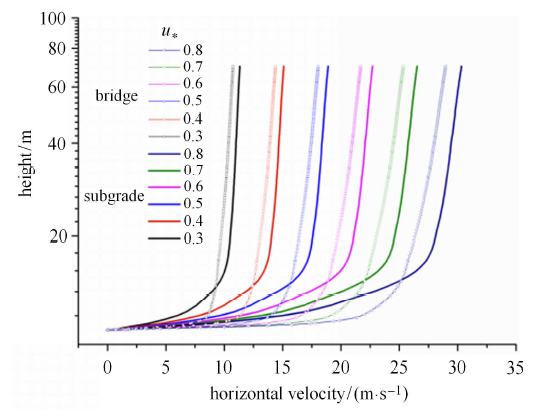Fig.7   The horizontal velocity profiles of wind field with height in the middle of railway at the position $x =170$m. Straight lines are the profiles in the subgrade design, and the shape lines are in the bridge design

### 图8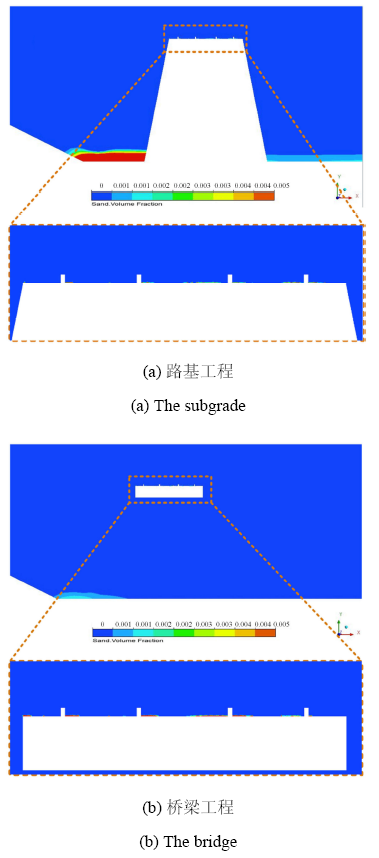Fig.8   The volume fraction of sand when the friction velocity is 0.3m/s with different design

### 图9Fig.9   The mass of sand accumulations on railway with friction velocity in two kinds of design forms

2015-2019年的4年间,如图10所示在敦煌台小里程方向路基工程范围(DK78+800-DK79+254.26)路基坡脚及路基面道碴间有大量积沙出现,因此2018年还对此段路基范围的积沙进行过清理;而在该桥敦煌台大里程方向(桥梁工程范围内DK79+254.26-DIK89+858.99)并未出现明显积沙,也未出现明显的风蚀现象. 从现场实际情况来看与本文的模拟结果吻合较好,也可以证实采用桥梁工程通过大风沙漠地区较之路基工程有着明显的优势.

### 图10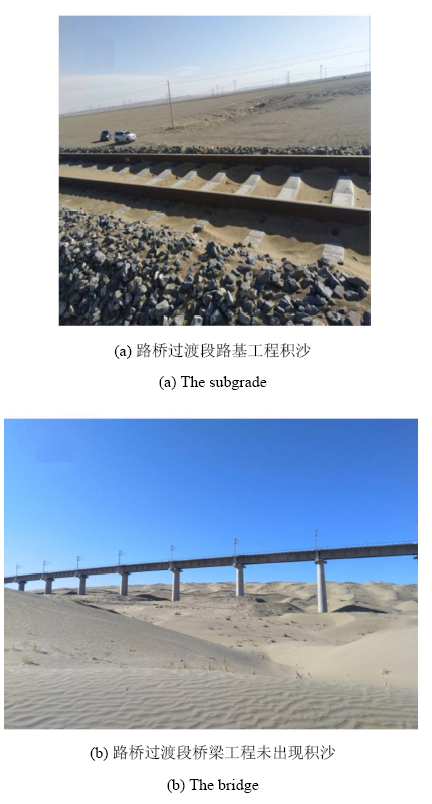Fig.10   Comparison of sand deposition between subgrade and bridge

## 3 结论

/

 〈〉